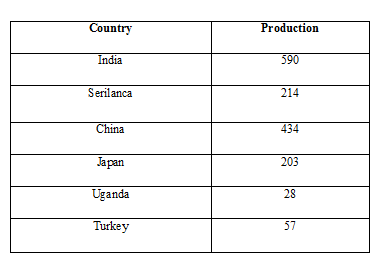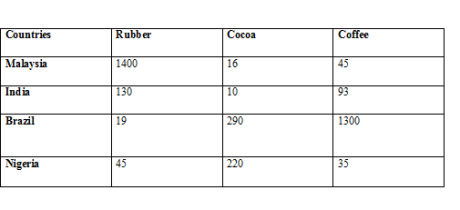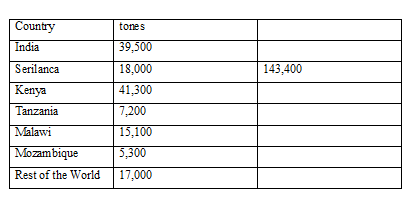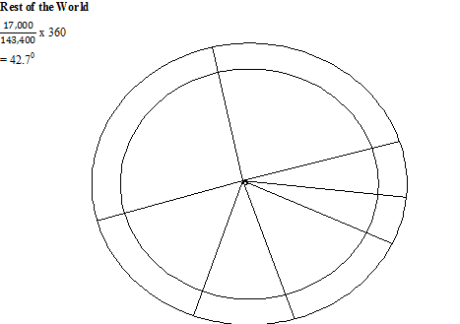• No products in the cart.

# GEO2/5: STATISTICS

## STATISTICS

It’s a representation of numerical information in form of graphs and diagrams and man maps.  This information can be represented using graphs, pie charts, maps.

Examples

-pictograms

-circles

-figurines.

Points to note

When drawing a statistical diagram it must

• Be drawn in pencil [sharp]

-Be meat

-Have a title

-Be accurate

-Have a scale if it is a graph

-Have both a scale and a key if it’s a map

-Have an appropriate size i.e. not too big or too small

-Use colors wherever possible and if it is a map they should be representing able

Be representative of the in forgiven i.e. you must use the most

GRAPHS

-Compound bar/line graph (divided bar)

-Divergence bar/line graph

-Population pyramids

-Circular graphs.

Note:

Any graphic representation always has an independent and dependent variable the dependent valuable is usually plotted on the vertical while the independent valuable is plotted on the horizontal axis.

SIMPLE BAR/LINE GRAPH

These are used mainly to represent a single value are a period of time. A bar graph will be used depending on the question.

-It’s easy to draw (not time-consuming)

-It’s easy to find a scale if the variables don’t vary a lot

-It’s easy to compare and contrast.

-It’s easy to interpret at a glance because one can easily see the periods of high or low production.

-It cannot be used if the dependent valuables are more than one. It can only be used if there is a single valuable.

-It may be hard to obtain a scale of the figures vary a lot.

Data

-The world’s production of tea is 1995.

-Heading producers in the countries (production countries)The data below shows the climate of AccraCOMPARATIVE GRAPHS/ GROUP GRAPHS

These are used to represent a number of variables in a year/a number of valuables over a period of time. They can also represent a number of valuables of various countries over a period of time.

COMPARATIVE BAR GRAPH/ GROUP BAR GRAPH

These ones represent the number of bars showing various items in a defined period of time.

– It’s easy to interpret because it clearly shows the periods of high and low production.

-A lot of information can be plotted on one graph.

-It’s easy to compare and contrast the data there for it has a good impression.

-It’s easy to draw/ consumes little time.

-Its time consuming if there is many bars and a lot of shading/overcrossing makes interpretation hard.

-It may be difficult to find the scale if the figures vary a lot.

-It may become overcrowded if there are many valuables over a period of time.It’s showing commercial crops of the world production into ones in 1985

-They can be used instead of bar

-They are similar to those of bar graph

-The line can crises cross making comparison difficult.

-The scale may be hard to find if figures are many and vary a lot

COMPOUND GRAPHS/ DIVIDED BAR/ LINE GRAPHS

They can be used instead of the group or comparative graphs when the data is in percentages they are more appropriate to use than the comparative graphs.

One can use either bars/ line graphs.

The graphs consume little space.

It’s easy to interpret.

It’s easy to draw.

It can create a lot of information on the same graph.

Since all the valuables do not begin at zero, it’s not easy to interpret at a glance.

The scale may be hard to find if the figures vary a lot.DIVERGENCY GRAPHS

It can either be a line or a graph.

It shows the divergences from the average

Procedure

Find the average of the figures given.

Subtract the average from each figure in order to find out the divergence.

Using the average as a starting point (zero line) finds an appropriate scale.

Plot the divergences.

The bar or line is easy to draw

It has a clear impression.

It’s easy to interpret.

It has a lot of tedious calculations.

It’s a time-consuming procedure.

Since the presentation is not in raw data (divergence) it may be hard to interpret.

It can only be used to represent one valuable.

Production of robuster coffee in 1982 in the major African countriesPOPULATION PYRAMIDS

They are used when representing the population structure. The population structure usually indicates population loagos according to age group and sex.

The bars are drawn horizontally and not verticallyDivided rectangles

These are rectangles at are drawn showing the data given. It looks like one block of a rectangle at have been subdivided. There is a simple divided rectangle of the compound divided rectangle.CIRCLES

DIVIDED CIRCLE (pie chart)

Note:

It’s suitably when the values don’t exceed eight.

Always start from the north moving to clockwise.

For better presentation draw an outer circle in which to include the information required.

The circle should be of an appropriate size.

WORLD TEA PRODUCTION IN TONES IN 1981India                                                   Kenya

x 360                                                 x 360

= 99.20                                                 =103.70

Serilanca                                            Tanzania

x 360                                      x 360

= 45.20                                                                 = 18.10

Marawi                                               Mozambique

x 360                                                  x 360

= 37.90                                                 = 13.30### ASSIGNMENT : STATISTICS AssignmentMARKS : 10  DURATION : 1 week, 3 days

SEE ALL
•YOU